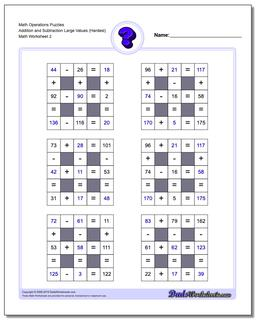PLEASE GO BACK AND USE THE BIG BLUE 'PRINT' BUTTON ON THE PAGE TO PRINT THE WORKSHEET CORRECTLY!Sorry for the trouble! The browser won't print the embedded worksheet PDF directly using the normal 'Print' command in the file menu, so you need to click the big 'Print' button to send just the worksheet and not the surrounding page to the printer.

# Math Worksheets: Number Grid Puzzles: Number Grid Puzzles: Math Operations Puzzles Addition and Subtraction Large Values (Hardest) (Second Worksheet)## Math Operations Puzzles Addition and Subtraction Large Values (Hardest) (Second Worksheet)

PropertyValue
DescriptionMath Operations Puzzles Addition and Subtraction Large Values (Hardest): Math logic puzzle worksheets that require students to fill in missing values. These worksheets have larger values for the operands and the sums than the ones above, and are appropriate for 4th grade students comfortable with larger problems. (Second Worksheet)
Resource TypeWorksheet